# Prime numberThe prime number 11 illustrated with square tiles. 12 squares can be arranged into a rectangle with sides of length 3 and 4, so 12 is not a prime number. There is no way to form a full rectangle more than one square wide with 11 squares, so 11 is a prime number.

A prime number is a whole number greater than 1 that can be evenly divided by only two different positive whole numbers, namely 1 and itself. The first few prime numbers are 2, 3, 5, 7, 11, 13, and 17.

A prime number p cannot be expressed as the product of two numbers a and b, i.e. as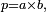$p=a\times b,$, except for the trivial factorizations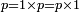$p = 1\times p = p\times 1$ that all numbers possess.

With the exception of 2, all prime numbers are odd numbers, but not every odd number is prime. For example,$9 = 3\times 3$ and$15 = 3\times 5,$ so neither 9 nor 15 is prime.

The study of prime numbers has a long history, going back to ancient times, and it remains an active part of number theory today. Although the study of prime numbers used to be an interesting but not terribly useful area of mathematical research, today it has important applications. Understanding properties of prime numbers and their generalizations is essential to modern cryptography — in particular to public key ciphers that are crucial to Internet commerce, wireless networks, and military applications. Less well-known is that other computer algorithms also depend on properties of prime numbers.

## Primes and factorization

The importance of prime numbers in arithmetic comes in large part from the unique factorization of numbers. Every number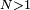$N > 1$ can be written as a product of prime factors, and all such expressions for any particular N will contain the same factors, differing only in the sequence in which they are listed.

For example, we can write$5040 = 7 \times 5 \times 3 \times 3 \times 2 \times 2 \times 2 \times 2$, where all of the factors in the product are prime numbers; there is no other way of writing 5040 as a product of prime numbers except by rearranging the prime factors we already have, such as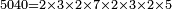$5040 = 2 \times 3 \times 2 \times 7 \times 2 \times 3 \times 2 \times 5$.

The existence of a single unique factorization into prime numbers is formalized as the Fundamental Theorem of Arithmetic. The fundamental property of the integers which it expresses is a critical building block in many of the important results in the area of elementary number theory. An analogy can be made between the role prime numbers play in arithmetic and the role atoms play in chemistry. 

It is this aspect of prime numbers that is of such interest to modern cryptography, in particular in the area of public key ciphers, as mentioned above. The most popular of these ciphers depends for its security on the difficulty of factoring extremely large numbers (many hundreds of digits in length) into their prime factors; were a fast algorithm for doing so to be discovered, some of today's most sophisticated ciphers would be instantly broken.

### Why 1 is not considered a prime

It might be intuitive to consider 1 a prime number, and in the past, mathematicians often did consider it to be a prime. The Fundamental Theorem of Arithmetic is a good example of why mathematicians have changed their minds on this point over the last century, and have definitively revised the formal definition of 'prime number' so that 1 is no longer included.

The Fundamental Theorem of Arithmetic is no longer strictly true if 1 is considered prime. Consider, for example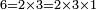$6 = 2\times 3 = 2\times 3\times 1$ - two different prime factorizations. Rather than adjust the formal definition of the Fundamental Theorem of Arithmetic, it is much easier to simply exclude 1 from the definition of the set of prime numbers.

This is an example of a more general trend in mathematics over the past century, which is to be much more careful in the treatment of 0 and 1 (which are very special numbers, with unusual properties that no other numbers have). Mathematicians need to be especially precise in statements that include them.

## There are infinitely many primes

One basic fact about the prime numbers is that there are infinitely many of them. In other words, the list of prime numbers 2, 3, 5, 7, 11, 13, 17, ... never stops. There are a number of ways of showing that there are infinitely many primes, but one of the oldest and most familiar proofs goes back go Euclid.

Euclid proved that for any finite set of prime numbers, there is always another prime number that is not in that set. Choose any finite set of prime numbers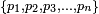$\{ p_1, p_2, p_3, \ldots, p_n \}$. Then the number$N = p_1 p_2 \cdots p_n +1$

presents a problem. On the one hand, the number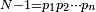$N-1 = p_1 p_2 \cdots p_n$ is a multiple of p1, and multiples of the same prime number are never next to each other, so N itself can't be a multiple of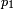$p_1$. The same argument actually shows that N itself cannot be a multiple of any of the prime numbers$p_1, p_2, p_3, \dots, p_n$. On the other hand, every number$N>1$ is divisible by some prime. (For example, its smallest divisor greater than 1 must be a prime.)

This shows that there is at least one prime number (namely, the smallest divisor of N greater than 1) that is excluded from our initial finite set. Since any finite set of prime numbers can thus be extended to a larger finite set of prime numbers, we conclude that there are infinitely many prime numbers.

Although most other proofs of the infinitude of prime numbers are more complicated, they can also provide more information. One mathematical milestone known as the Prime Number Theorem estimates how many of the numbers between 1 and x are prime numbers (see below). Another such proof is Euler's demonstration that the sum of the reciprocals of the primes diverges to ∞.

## Locating primes

How can we tell which numbers are prime and which are not? It is sometimes possible to tell that a number is not prime by looking at its digits: for example, any number whose last digit is even is divisble by 2, and any number ending with 5 or 0 is divisible by 5. Therefore, except for the prime numbers 2 and 5, any prime number must end with the digit 1, 3, 7, or 9. This check can be used to rule out the possibility of a randomly chosen number being prime more than half of the time, but numbers that end with 1, 3, 7, or 9 often have divisors that are harder to spot.

To find large prime numbers, we must use a systematic procedure — an algorithm. Nowadays, prime-finding calculations are performed by computers, often using very complicated algorithms, but there are simple algorithms that can be carried out by hand if the numbers are small. In fact, the simplest methods for locating prime numbers are some of the oldest algorithms, known since antiquity. Two classical algorithms are called trial division and the sieve of Eratosthenes.

### Trial division

Trial division consists of systematically searching the list of numbers$2, 3, \dots, n-1$ for a divisor; if none is found, the number is prime. If n has a small divisor, we can quit as soon as we've found it, but in the worst case — if n is prime — we have to test all$n-2$ numbers to be sure. This algorithm can be improved by realizing the following: if n has a divisor a that is larger than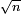$\scriptstyle\sqrt n$, there must be another divisor b that is smaller than$\sqrt n$. Thus, it is sufficient to look for a divisor up to$\sqrt n$. This makes a significant difference: for example, we only need to try dividing by 2, 3, ..., 31 to verify that 997 is prime, rather than all the numbers 2, 3, ..., 996. Trial division might be described as follows using pseudocode:

Algorithm: trial division

Given n,
For each i = 2, 3, ... less than or equal to$\sqrt{n}$:
If i divides n:
Return "n is not prime"
Else:
Continue with the next i
When all i have been checked:
Return "n is prime"

### Sieve of Eratosthenes

The Sieve of Eratosthenes not only provides a method for testing a number to see if it is prime, but also for enumerating the (infinite) set of prime numbers. The idea of the method to write down a list of numbers starting from 2 ranging up to some limit, say:

2, 3, 4, 5, 6, 7, 8, 9, 10, 11, 12, 13, 14, 15, 16, 17, 18, 19, 20

The first number (2) is prime, so we mark it, and cross out all of its multiples:

2, 3, 4, 5, 6, 7, 8, 9, 10, 11, 12, 13, 14, 15, 16, 17, 18, 19, 20

The smallest unmarked number is 3, so we mark it and cross out all its multiples (some of which may already have been crossed out):

2, 3, 4, 5, 6, 7, 8, 9, 10, 11, 12, 13, 14, 15, 16, 17, 18, 19, 20

The smallest unmarked number (5) is the next prime, so we mark it and cross out all of its multiples:

2, 3, 4, 5, 6, 7, 8, 9, 10, 11, 12, 13, 14, 15, 16, 17, 18, 19, 20

Notice that there are no multiples of 5$(\le 20)$ that have not already been crossed out, but that doesn't matter at this stage. Proceeding as before, we add 7, 11, 13, 17 and 19 to our list of primes:

2, 3, 4, 5, 6, 7, 8, 9, 10, 11, 12, 13, 14, 15, 16, 17, 18, 19, 20

We have now found all prime numbers up to 20.

## Distribution of prime numbers

It is evident that the prime numbers are randomly distributed but, unfortunately, we don't know what 'random' means. — R. C. Vaughan

The list of prime numbers suggests that they thin out the further you go: 44% of the one-digit numbers are prime, but only 23% of the two-digit numbers and 16% of the three-digit numbers. The trial division method explained above provides an intuitive explanation. To test whether a number n is prime, you have to try whether it can be divided by all numbers between 2 and$\scriptstyle\sqrt n$. Large numbers have to undergo more tests, so fewer of them will be prime.

The Prime Number Theorem explains how fast the prime numbers thin out. It says that if you are looking around the number n, about one in every$\scriptstyle\log\, n$ numbers is prime (here,$\scriptstyle\log\, n$ denotes the natural logarithm of n). The formal statement of the prime number theorem is$\lim_{x\to\infty} \frac{\pi(x) \log x}{x} = 1$

where π(x) is the number of primes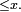$\le x.$

## Some unsolved problems

There are many unsolved problems concerning prime numbers. A few such problems (posed as conjectures) are:

### The twin prime conjecture

Twin primes are pairs of prime numbers differing by 2. Examples of twin primes include 5 and 7, 11 and 13, and 41 and 43. The Twin Prime Conjecture states that there are an infinite number of these pairs.

### The Goldbach conjecture

The Goldbach conjecture states that every even number greater than 2 can be expressed as the sum of two primes. For example, if you choose the even number 48, you can find 48 = 41 + 7 where 41 and 7 are prime numbers.

### Primes of special forms

Prime numbers having special forms are important in various applications. For instance, Fermat primes are prime numbers that can be written as$2^{2^n}+1$ for some positive integer n. Such prime numbers make a surprising appearance in the solution to the problem of which regular polygons can be constructed with a straight-edge and compass. Many unsolved problems ask whether there are infinitely many primes of a certain form. For instance, it is unknown if there are infinitely many Fermat primes, Mersenne primes, or Sophie-Germain primes. See the Related articles subpage for a list of special types of primes.

## Alternative definition

A prime number is usually defined as a positive integer other than 1 that is (evenly) divisible only by 1 and itself. However, there is another way of defining prime numbers, and that is the observation that a number p is prime if in all cases where it can evenly divide the product of two numbers, a and b, it must also evenly divide one of those two numbers.

A simple counter-example is that 4 evenly divides 12 (i.e. is a factor of 12), but 4 does not divide 2 and 4 does not divide 6 even though 12 is 2 times 6. This means that 4 is not a prime number.

This example illustrates the principle at work in this definition; when the number p cannot evenly divide one of the two numbers, either a or b, it must be made up of two factors, one of which divides one of the numbers (a), and the other the second number (b).

If the first characterization of prime numbers is taken as the definition, the second is derived from it as a theorem (known as Euclid's lemma), and vice versa.

We may express this second possible definition in mathematical notation as follows: A number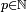$p \in \mathbb{N}$ (natural number) is prime if for any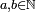$a, b \in \mathbb{N}$ such that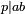$p | ab$ (read p divides ab), either p | a or p | b.

It may feel natural to take the first of the two characterizations we gave as the definition. However, when one generalizes the concept of "integer", and starts considering algebraic integers, it becomes clear that, while one can study elements that are divisible only by units and (unit multiples of) themselves, these elements need not have all of the habitual properties of prime numbers. Thus, it has become customary to call such elements irreducible, and to reserve the adjective prime for prime ideals, which are objects whose definition mimics the second characterization of prime numbers given above. (Algebraic integers factorize uniquely into prime ideals, but not, in general, into irreducibles; this is the main reason why prime ideals deserve the label prime in their name, whereas irreducibles are deprived of it.)

There are rings -- including the "usual" integers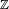$\mathbb{Z}$ -- in which all irreducible elements generate prime ideals, and all prime ideals are generated by irreducible elements. (In$\mathbb{Z}$ and a few other rings, the proof of this statement is based on the fact that the remainder of a division is smaller than the divisor. Rings for which this is true are called Euclidean domains.) In many rings, however, irreducible elements may generate non-prime ideals, and a prime ideal may fail to be generated by any irreducible element (or by any one element of the ring).

## References and notes

1. In mathematics, a product is a quantity (amount) obtained by multiplication of two numbers: "the product of 2 and 5 is 10"; "the product of 4 and 6 is 24".
2. This analogy means that the atoms of an element are the smallest possible components of that substance just as prime numbers are the least possible factors of a number. Divide them any smaller and the element no longer exists. Water, H2O, can be divided into its components, or "products", of H and two O, 1 hydrogen and 2 oxygen atoms. These are like the prime number factors. As elements, these simply can not be divided into anything smaller. This is not unlike the prime number products.
3. Topic: 1 as a prime number; see in particular the comments by John Conway.
4. The number N has at least one divisor greater than 1, because N is a divisor of itself. Let q denote the smallest divisor of N greater than 1. To see why q must be prime: Any divisor of q is also a divisor of N, and since q is the smallest, then q has no divisors smaller than itself except 1, therefore it is prime.Some content on this page may previously have appeared on Citizendium; see the talk page.